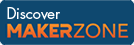Cody

# Problem 10. Determine whether a vector is monotonically increasing

Created by Cody Team in Community

Return true if the elements of the input vector increase monotonically (i.e. each element is larger than the previous). Return false otherwise.

Examples:

``` Input  x = [-3 0 7]
Output tf is true```
``` Input  x = [2 2]
Output tf is false```

### Solution Stats

27.93% Correct | 72.07% Incorrect
Last solution submitted on Mar 25, 2019

#### TagsMATLAB and Simulink resources for Arduino, LEGO, and Raspberry Pi